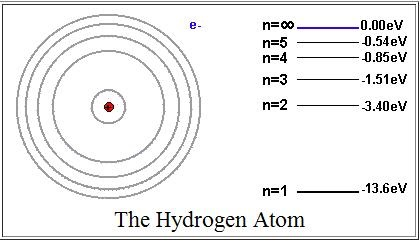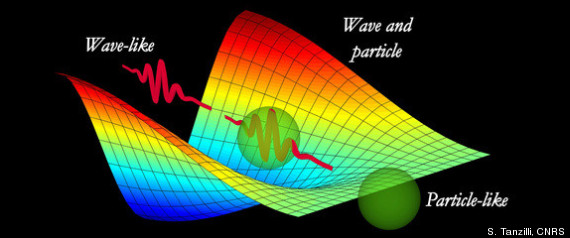Electricity and Magnetism

# E+M Warmups: Level 2 Challenges

Researchers looking for dark matter signals like to use Roman lead, in particular the lead found in old Roman shipwrecks, which has caused tension between physicists and archaeologists. The reason Roman lead is valuable is that Roman lead is less radioactive than recently mined lead. Lead is primarily used in particle physics experiments as a shielding agent, in that it prevents outside particles from entering a detector and giving false signals. If the lead is radioactive though, the decay of the lead itself will generate noise in the detector. Mined lead is partly made of $^{210}Pb$, which has a half-life of 22.2 years. If Roman lead was mined 1713 years ago, what is the ratio of the amount of $^{210}Pb$ in the Roman lead now versus when it was mined?Recordings that get played speakers can be very detailed, consisting of tens or hundred of distinct sound sources, each of which contribute a continuum of frequency components. Yet there is only one speaker. How is it possible for a single speaker to faithfully reproduce complex sounds?In atoms, electrons jump between energy levels, and they absorb or radiate energy in the form of light (photons). An electron falls to a lower energy level when it radiates a photon, and jumps to a higher energy level when it absorbs a photon.

Find the wavelength of the photon that radiates when an electron jumps from the $n = 3$ energy level down to the $n = 2$ energy level. Where is this photon in the electromagnetic spectrum?

Assumptions

• $h$ (Planck's constant) = $4.14 \times 10^-15$ eV s.
• $c$ (Speed of light) = $3 \times 10^8$ m/s.
• All values are approximate.
• Assume that this is a hydrogen atom.Suppose that an infinitely long straight current-carrying wire has a uniform linear charge density $\lambda.$ Let this wire be on the $y$-axis of the $xy$-plane, and let $x > 0$ be the distance between the $y$-axis and a point $P$ on the $xy$-plane. What is the strength of the electric field at the point $P?$

Note: $\epsilon_0$ in the choices below denotes the electric constant.An electron, practically at rest is initially accelerated through a potential difference $100 \text{ V}$. It then has a de Broglie wavelength of $x_1$.

It then gets retarded through $19 \text{ V}$ and has a wavelength of $x_2$.

A final retardation through $32 \text{ V}$ changes the wavelength to $x_3$.

What is $\displaystyle\frac{x_3-x_2}{x_1}$ as a percentage?

×